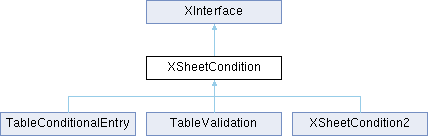LibreOffice LibreOffice 7.4 SDK API Reference
XSheetCondition Interface Referencepublished

provides methods to access the settings of a condition in a conditional format or data validation. More...

`import"XSheetCondition.idl";`

Inheritance diagram for XSheetCondition:## Public Member Functions

com::sun::star::sheet::ConditionOperator getOperator ()
returns the operator in the condition. More...

void setOperator ([in] com::sun::star::sheet::ConditionOperator nOperator)
sets the operator in the condition. More...

string getFormula1 ()
returns either the comparison value, which is used in the condition, or the first value if two values are needed for the operator. More...

void setFormula1 ([in] string aFormula1)
sets either the comparison value, which is used in the condition, or the first value if two values are needed for the operator. More...

string getFormula2 ()
if two values are needed for the operator, this method returns the second one. More...

void setFormula2 ([in] string aFormula2)
if two values are needed for the operator, this method sets the second one. More...

returns the position in the document which is used as a base for relative references in the formulas. More...

sets the position in the document which is used as a base for relative references in the formulas. More...Public Member Functions inherited from XInterface
any queryInterface ([in] type aType)
queries for a new interface to an existing UNO object. More...

void acquire ()
increases the reference counter by one. More...

void release ()
decreases the reference counter by one. More...

## Detailed Description

provides methods to access the settings of a condition in a conditional format or data validation.

com::sun::star::sheet::TableConditionalEntry
com::sun::star::sheet::TableValidation

## ◆ getFormula1()

 string getFormula1 ( )

returns either the comparison value, which is used in the condition, or the first value if two values are needed for the operator.

Returns
a formula, a numeric constant, or a string constant in quotes.

## ◆ getFormula2()

 string getFormula2 ( )

if two values are needed for the operator, this method returns the second one.

Returns
a formula, a numeric constant, or a string constant in quotes.

## ◆ getOperator()

 com::sun::star::sheet::ConditionOperator getOperator ( )

returns the operator in the condition.

## ◆ getSourcePosition()

returns the position in the document which is used as a base for relative references in the formulas.

## ◆ setFormula1()

 void setFormula1 ( [in] string aFormula1 )

sets either the comparison value, which is used in the condition, or the first value if two values are needed for the operator.

Parameters
 aFormula1 a formula, a numeric constant, or a string constant in quotes.

## ◆ setFormula2()

 void setFormula2 ( [in] string aFormula2 )

if two values are needed for the operator, this method sets the second one.

Parameters
 aFormula2 a formula, a numeric constant, or a string constant in quotes.

## ◆ setOperator()

 void setOperator ( [in] com::sun::star::sheet::ConditionOperator nOperator )

sets the operator in the condition.

## ◆ setSourcePosition()

 void setSourcePosition ( [in] com::sun::star::table::CellAddress aSourcePosition )

sets the position in the document which is used as a base for relative references in the formulas.

The documentation for this interface was generated from the following file: